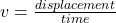## Calculate the speed of a car if it covers the distance of 100m in 5seconds​.

Question

Calculate the speed of a car if it covers the distance of 100m in 5seconds​.

in progress 0
5 months 2021-08-09T07:56:28+00:00 2 Answers 3 views 0

200m/s

Explanation:Explanation:

distance =100m

time=5seconds

speed=?

so distance/time

=100/5

=20